Next: About this document Up: Computational Methods in Finance Previous: Duality and Qualitative Properties

# Dynamic Programming

Dynamic programming is a method for valuing American style options and other financial instruments that allow the holder to make decisions that effect the ultimate payout. The idea is to define the appropriate value function, f(x,t), that satisfies a nonlinear version of the backwards evolution equation (7). I will explain the idea in a simple but somewhat abstract situation. From in the previous section, it is possible to use these ideas to treat other related problems.

We have a Markov chain as before, but now the transition probabilities depend on a ``control parameter'',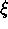. That is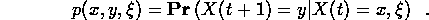In the ``stochastic control problem'', we are allowed to choose the control parameter at time t,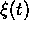, knowing the value of X(t) but not any more about the future than the transition probabilities. Because the system is a Markov chain, knowledge of earlier values, X(t-1),, will not help predict or control the future. Choosingas a function of X(t) and t is called ``feedback control'' or a ``decision strategy''. The point here is that the optimal control policy is a feedback control. That is, instead of trying to choose a whole control trajectory,for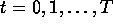, we instead try to choose the feedback functions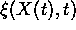. We will write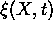for such a decision strategy.

Any given strategy has an expected payout, which we write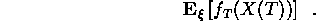Our object is to compute the value of the financial instrument under the optimal decision strategy: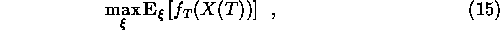and the optimal strategy that achieves this.

The appropriate collection of values for this is the ``cost to go'' function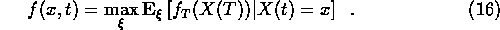As before, we have ``initial data''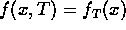. We need to compute the values f(x,t) in terms of already computed values f(x,t+1). For this, we suppose that the optimal decision strategy at time t is not yet known but those at later times are already computed. If we use control variableat time t, and the optimal control thereafter, we get payout depending on the state at time t+1: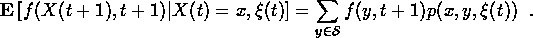Maximizing this expected payout overgives the optimal expected payout at time t: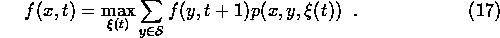This is the principle of dynamic programming. We replace the ``multiperiod optimization problem'' (11) with a sequence of hopefully simpler ``single period'' optimization problems (13) for the cost to go function.Next: About this document Up: Computational Methods in Finance Previous: Duality and Qualitative Properties

Jonathan Goodman
Tue Sep 15 17:12:32 EDT 1998